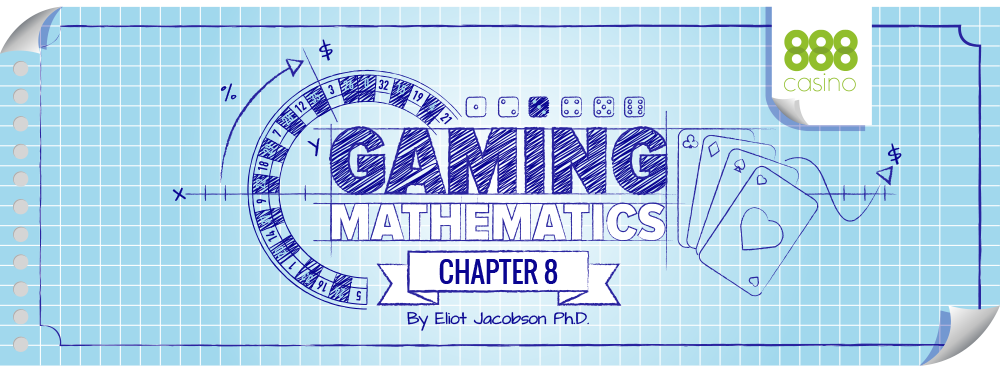# PROBABILITY

The backbone of casino game mathematics is “probability.” Informally, we understand probability as a number that describes the chance that something will occur. It is usually given as a fraction or decimal with a value between 0 and 1, or as a percent with value between 0% and 100%. A probability of 0 means the event can never occur. A probability of 1 means the event always occurs. For example, toss two dice and have the sum come up 13; that’s impossible, so the probability is 0. Toss a coin and have it come up either heads or tails; that’s a certainty, so the probability is 1. Dice and coins never land on edge in our mathematically perfect world.

The formal theory of probability begins by understanding what’s known as the “sample space.” This is simply a description of all possible outcomes – everything that can possibly happen. Some examples: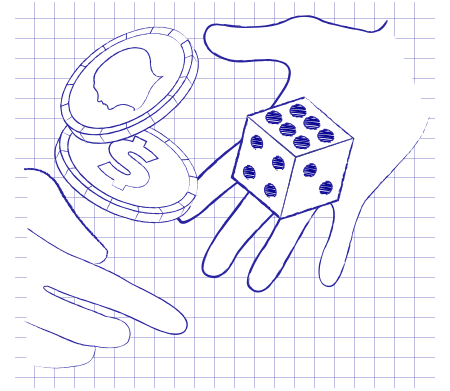1. There are 2 outcomes when a coin is tossed; the sample space is {Heads, Tails}.

2. There are 6 outcomes when a single dice is rolled; the sample space is {1, 2, 3, 4, 5, 6}.

3. There are 36 outcomes when two dice are rolled (the first dice and the second dice each produce a value from 1 to 6, so there are 6 × 6 = 36 outcomes). The sample space is {[1,1], [1,2], [1,3], [1,4], [1,5], [1,6], [2,1], … and so on}, or more simply, using set builder notation, it is {[x,y] | 1 ≤ x ≤ 6 , 1 ≤ y ≤ 6 }.

4. There are 38 outcomes when a live roulette wheel is spun. The sample space consists of the numbers 1 through 36, together with zero and double-zero.

5. There are 52 ways a single card can be dealt from a deck. The sample space consists of the set of card values (rank and suit).

6. There are 1,326 two-card starting hands in Texas Hold’ em. The sample space consists of the set of pairs of cards.

Many experiments have a sample space that is easily understood from the nature of the experiment but may not be explicitly described. Sample spaces are often very large for casino games, reflecting the intuitive feeling that there are too many things that can happen to count them all. Mathematicians have to count them all.

* There are 66,300 ways a player can be dealt two cards against a dealer up-card in single deck blackjack.
* There are 2,598,960 ways that 5 cards can be dealt to a player in poker.
* There are 407,170,400 ways that the player and dealer can be dealt their respective three cards hands in Three Card Poker.
* There are 55,627,620,048,000 ways two players can play out a hand of Texas Hold’ em, including their initial two cards, the three flop cards, the turn and the river, playing head’s up against each other.

An “event” consists of some of the things that can happen in the experiment. An event is a way of describing some of the things out of everything that might happen in a game. Here are some examples of events that correspond to some of the games in the list above:

* Tossing a coin and getting heads.
* Rolling two dice and getting a sum of 7.
* Being dealt a live blackjack against a dealer up-card of an Ace.
* Being dealt a pat full house as a five card poker hand.
* Being dealt a straight that loses to a higher straight in Three Card Poker.
* Being dealt a pocket pair in Texas Hold’em.

To compute the probability of an event we need to know two pieces of information. First, we need a full count of the number of individual elements in the sample space. Second, we need to know how many individual elements are in the collection that corresponds to the event. Simply put, we need to know the size of the sample space and the size of the event.

## KNOWING THESE VALUES, WE DEFINE THE PROBABILITY OF THE EVENT BY THE EQUATION: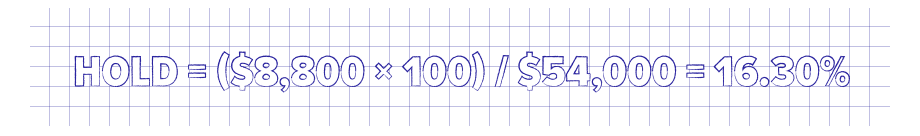The word “Probability” is cumbersome to write out; it is customary to use the letter “P” when referring to the probability of an event.

What is not evident by the equation for probability is how to count the size of various collections. Unfortunately, in gaming there are very few simple problems and these counting problems can be exceedingly complex. For those cases when counting is easy, probabilities can be quickly computed. We’ll go through several examples to demonstrate some of the techniques. Hopefully these examples will help clarify the concept of probability for casino games and some of the methods that are used to come up with these values.

## TOSSING A COIN PROBABILITY

When tossing a coin, let H = “Heads” and T = “Tailes.” For a single coin toss, the sample space is {H, T} and it has two elements in it. To get a “heads” corresponds to the event {H}, which has a single element in it. So:

P(heads) = 1 / 2 = 0.5000.

## TEXAS HOLD’EM PROBABILITIES FIRST & SECOND DEALING CARDS

In Texas Hold’em, there are 52 possible first cards you can be dealt and 51 possible second cards you can be dealt. Because the order of the cards doesn’t matter, we divide by 2 to take account of symmetry. The size of the sample space is:

(52 × 51) / 2 = 1326.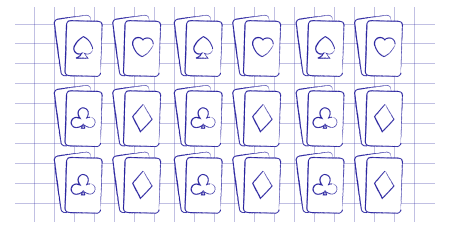## POCKET PAIR

We consider the event of being dealt a pair. If we look at pairs of deuces, there are six possible pairs (again, order doesn’t matter): {[2C,2D], [2C,2H], [2C,2S], [2D,2H], [2D,2S], [2H,2S]}. For any rank of card, there are six possible pairs of that rank. There are 13 possible ranks for the pair, and six ways of making the pair at that rank. This gives 13 × 6 = 78 pairs. So, the size of the event of being dealt a pocket pair is 13 × 6 = 78. So:

P(pocket pair) = 78 / 1326 = 0.0588.## ROLLING DICE PROBABILITY

When rolling two dice, the sample space is {[x,y] | 1 ≤ x ≤ 6 , 1 ≤ y ≤ 6 }; the sample space has 36 elements in it. The event “getting a sum of 7” corresponds to the subset of the sample space {[1,6], [2,5], [3,4], [4,3], [5,2], [6,1]}. This subset has 6 elements in it. Therefore:

P(sum of 7) = 6 / 36 = 0.1667.

This figure gives the probabilities for the various sums of two dice:

## BLACKJACK VS. DEALER ACE PROBABILITYIn single deck blackjack, there are (52 × 51) / 2 = 1,326 possible two card starting hands for the player. For each such hand, there are 50 cards left in the deck that can be dealt as the up-card for the dealer. Thus there are 1,326 × 50 = 66,300 possible starting situations. So the sample space has size 66,300. The event we’re interested in is being dealt a blackjack against a dealer Ace. A player blackjack consists of a face card (16 of them) and an Ace (4 of them). There are 16 × 4 = 64 ways for the player to be dealt a blackjack. There are 3 remaining aces out of 50 cards for the dealer up-card. So there are 16 × 4 × 3 = 192 ways for the player to have a blackjack against a dealer up-card of an Ace. The event of a player blackjack against a dealer Ace has size 192. Therefore:

P(Blackjack vs. Dealer Ace) = 192 / 66,300 = 0.002896.

This means that the situation where a player can consider taking “even money” comes up about 29 times in every 10,000 hands, or about once every 345 hands. At a full table and at standard dealing speeds, this amounts to about one player “even money” decision per table per hour in single deck.
Doing the same computations for a six-deck shoe game, we get a sample space with size 15,039,960 and an event with size 52,992, so that:

P(Blackjack vs. Dealer Ace) = 52,992 / 15,039,960 = 0.003523.

This means that even money situations come up about 35 times per 10,000 hands, or about once every 284 hands. Even money is far more common, and therefore potentially far more profitable for the casino, in a shoe game.

## THREE CARD PROBABILITIES

In this example we are going to go through the mathematical details of the Pair Plus bet in Three Card Poker and compute all the probabilities.

First of all, the sample space consists of all the distinct three card hands that can be dealt from a deck of 52 playing cards. There are 52 possible values for the first card, 51 possible values for the second card, and 50 possible values for the third card. Three cards can be rearranged in six possible ways, but still be the same three cards: ABC, ACB, BAC, BCA, CAB and CBA. Therefore, the number of distinct three card hands is:

52 × 51 × 50) / 6 = 22,100.

## EVENT: STRAIGHT FLUSH

These are easy to count. In order to have a straight, we have to get one of the hands A23, 234, 345, 456, 567, 678, 789, 89T, 9TJ, TJQ, JQK, QKA in one of the four suits. There are 12 straights and each straight can happen in one of the four suits, so there are 12 × 4 = 48 ways to get a straight flush. So:

P(Straight Flush) = 48 / 22,100 = 0.002172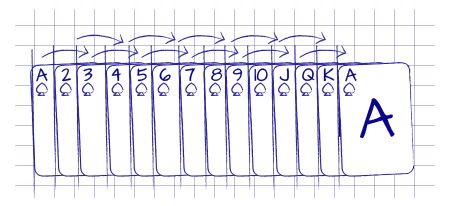## EVENT: THREE OF A KIND

Again, we can count these directly. For deuces, we can get trips with any of the hands [2C,2D,2H], [2C,2D,2S], [2C,2H,2S] and [2D,2H,2S]. There are four trips for any rank and there are thirteen ranks, so there are 13 × 4 = 52 ways to get three of a kind. So:

P(Three of a Kind) = 52 / 22,100 = 0.002353.## EVENT: STRAIGHT (NOT STRAIGHT FLUSH)

We begin by counting all straights, including the straight flushes. As above, a straight consists of one of the hand types A23, 234, 345, 456, 567, 678, 789, 89T, 9TJ, TJQ, JQK, QKA. Let’s look at A23 in particular. We can have any suits for the A, 2 and 3; there are four choices for the suit of each (clubs, diamonds, hearts and spades). Therefore the A23 straight consists of picking a card from each of {AC, AD, AH, AS}, {2C, 2D, 2H, 2S} and {3C, 3D, 3H, 3S}. There are 4 × 4 × 4 = 64 ways of choosing these three cards. So there are 64 straights of type A23. There are 12 types of straights and each straight can happen in 64 ways. So the total number of straights is 12 × 64 = 768. But this number includes the 44 straight flush hands that we already counted, so we have to subtract those. This leaves 768 – 48 = 720 straights that are not a straight flush. So:

P(Straight) = 720 / 22,100 = 0.032579.

## EVENT: FLUSH (NOT STRAIGHT FLUSH)

We need to count all the hands XYZ where X, Y and Z have the same suit and the hand is not a straight flush. We’ll pick on the suit Clubs. There are 13 cards. We can choose any of these 13 for the first card, any of the remaining 12 for the second card, and any of the remaining 11 for the third card. Because three cards can be rearranged in six possible ways and the order the cards were dealt doesn’t matter, it follows that the number of ways of getting a flush in clubs is (13 × 12 × 11) / 6 = 286. Of these flushes, 12 of them are a straight flush. This leaves 286 – 12 = 274 flushes in clubs that aren’t straight flushes. Because there are four suits, it follows that the total number of flushes is 274 × 4 = 1,096. So:

P(Flush) = 1,096 / 22,100 = 0.049593

## EVENT: PAIR.

There are 6 ways of getting a pair of any rank. For example, to get a pair of deuces the player must hold one of the pairs [2C,2D], [2C,2H], [2C,2S], [2D,2H], [2D,2S] or [2H,2S]. Once the pair is dealt, the third card has to be a card of a different rank (otherwise the hand would be trips). There are 48 cards that are not the same rank as the pair. Putting this together, there are 13 possible ranks for the pair, 6 pairs of that rank, and 48 possible third cards of a different rank. Multiplying these together, we get a total of 13 × 6 × 48 = 3,744 pairs. So:

P(Pair) = 3,744 / 22,100 = 0.169412.

## EVENT: NOTHING

This final case is the easiest. To get nothing simply means that the hand isn’t any of the above. We simply subtract all of the results above from the total number of hands. This gives 22,100 – 48 – 52 – 720 – 1,096 – 3,744 = 16,440 hands that are losing hands for the Pair Plus wager. So:

P(Nothing) = 16,440 / 22,100 = 0.743891.

## HIT FREQUENCY

We now illustrate how to compute the hit frequency. For the Pair Plus wager, a player wins any time he has a pair or better. The hit frequency corresponds to the probability of being dealt a pair or better. To find the number of elements in this event, we simply add up all the possible winning hands. Doing this, we get 48 + 52 + 720 + 1,096 + 3,744 = 5,660. So:

Hit Frequency = P(Winning) = 5,660 / 22,100 = 0.256109.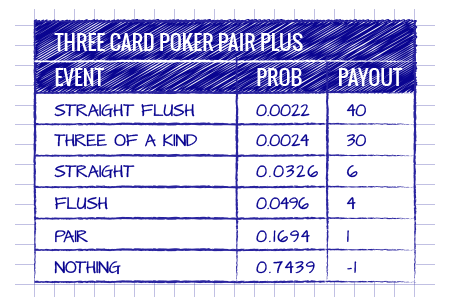It follows that the hit frequency is 1-in-3.9. Rounding off, the player wins about once every four times he makes a Pair Plus wager.

Finally, note that if we add all the probabilities together, we get 0.002172 + 0.002353 + 0.032579 + 0.049593 + 0.169412 + 0.743891 = 1.000000. This simply means that the probability that something is going to happen is 1, a certainty.

The following figure summarizes the probabilities we just computed and the payouts for the various events using the most generous pay table Shuffle Master offers for this wager. It is customary to indicate a payout of -1 when the player loses; after all, he lost his wager. In all other cases, the player’s wager is returned and he is paid the amount indicated in the table.